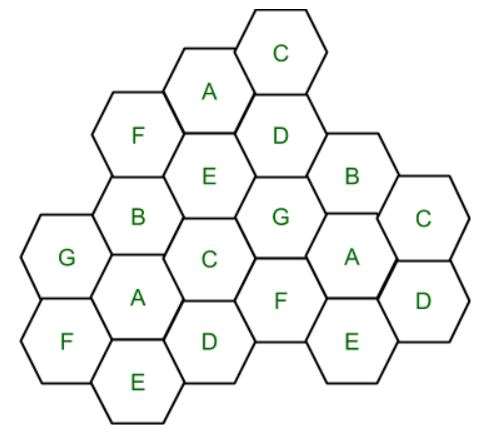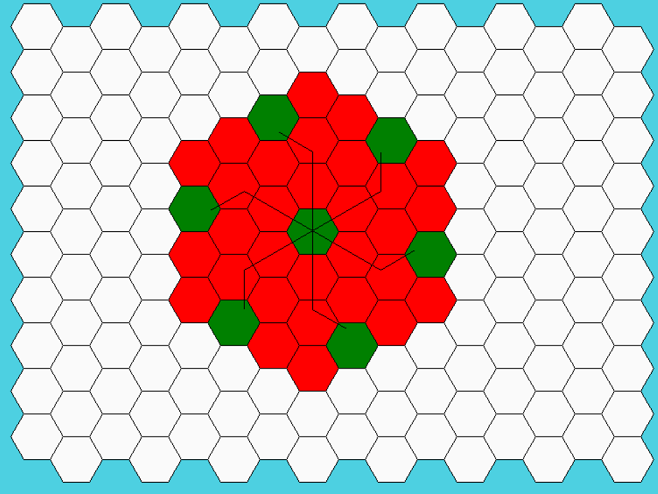GFG App
Open AppBrowser
Continue

Frequency Reuse is the scheme in which allocation and reuse of channels throughout a coverage region is done. Each cellular base station is allocated a group of radio channels or Frequency sub-bands to be used within a small geographic area known as a cell. The shape of the cell is Hexagonal. The process of selecting and allocating the frequency sub-bands for all of the cellular base station within a system is called Frequency reuse or Frequency Planning. Salient features of using Frequency Reuse:

• Frequency reuse improve the spectral efficiency and signal Quality (QoS).
• Frequency reuse classical scheme proposed for GSM systems offers a protection against interference.
• The number of times a frequency can be reused is depend on the tolerance capacity of the radio channel from the nearby transmitter that is using the same frequencies.
• In Frequency Reuse scheme, total bandwidth is divided into different sub-bands that are used by cells.
• Frequency reuse scheme allow WiMax system operators to reuse the same frequencies at different cell sites.Cell with the same letter uses the same set of channels group or frequencies sub-band. To find the total number of channel allocated to a cell: S = Total number of duplex channels available to use k = Channels allocated to each cell (k<S) N = Total number of cells or Cluster Size Then Total number of channels (S) will be,

```S = kN

Frequency Reuse Factor = 1/N```

In the above diagram cluster size is 7 (A,B,C,D,E,F,G) thus frequency reuse factor is 1/7. N is the number of cells which collectively use the complete set of available frequencies is called a Cluster. The value of N is calculated by the following formula:

`N = I2 + I*J + J2 `

Where I,J = 0,1,2,3… Hence, possible values of N are 1,3,4,7,9,12,13,16,19 and so on. If a Cluster is replicated or repeated M times within the cellular system, then Capacity, C, will be,

`C = MkN = MS`

In Frequency reuse there are several cells that use the same set of frequencies. These cells are called Co-Channel Cells. These Co-Channel cells results in interference. So to avoid the Interference cells that use the same set of channels or frequencies are separated from one another by a larger distance. The distance between any two Co-Channels can be calculated by the following formula:

` D = R * (3 * N)1/2`

Where, R = Radius of a cell N = Number of cells in a given cluster

• Improved Spectral Efficiency: By reusing the same frequency in different geographic areas, spectral efficiency can be improved, enabling more efficient spectrum usage.
• Better Quality of Service: With the ability to reuse the same frequency in different cells, the interference between cells can be minimized, leading to better quality of service.
•  Cost-Effective: Frequency reuse can reduce the cost of building a cellular network since fewer frequency bands are required.
Scalability: Frequency reuse enables the network to be easily scaled by adding more cells as needed.
• Increased Network Capacity: Frequency reuse allows more cells to be served with the same amount of spectrum, resulting in increased network capacity.
• Scalability: Frequency reuse enables the network to be easily scaled by adding more cells as needed.

• Increased Interference: Frequency reuse can result in increased interference, particularly in areas where cells are closely spaced. This can reduce the quality of service and network capacity.
• Implementation Complexity: Frequency reuse requires careful planning to ensure that cells are appropriately spaced and that interference is minimized. This can make the implementation process more complex and time-consuming.
• Reduced Coverage: With the use of smaller cells to achieve higher capacity, the coverage area of each cell is reduced, requiring more base stations and infrastructure.
• Increased Power Consumption: Due to the use of smaller cells, more base stations are required, leading to higher power consumption and operational costs.
• Increased Network Cost: The cost of implementing a frequency reuse system may be higher due to the need for additional infrastructure and careful planning to ensure proper frequency reuse.

Below is the Python code for visualizing the Frequency Reuse concept

## Python3

 `#!/usr/bin/python`   `from` `math ``import` `*`   `# import everything from Tkinter module` `from` `tkinter ``import` `*`   `# Base class for Hexagon shape` `class` `Hexagon(``object``):` `    ``def` `__init__(``self``, parent, x, y, length, color, tags):` `        ``self``.parent ``=` `parent` `        ``self``.x ``=` `x` `        ``self``.y ``=` `y` `        ``self``.length ``=` `length` `        ``self``.color ``=` `color` `        ``self``.size ``=` `None` `        ``self``.tags ``=` `tags` `        ``self``.draw_hex()`   `    ``# draw one hexagon` `    ``def` `draw_hex(``self``):` `        ``start_x ``=` `self``.x` `        ``start_y ``=` `self``.y` `        ``angle ``=` `60` `        ``coords ``=` `[]` `        ``for` `i ``in` `range``(``6``):` `            ``end_x ``=` `start_x ``+` `self``.length ``*` `cos(radians(angle ``*` `i))` `            ``end_y ``=` `start_y ``+` `self``.length ``*` `sin(radians(angle ``*` `i))` `            ``coords.append([start_x, start_y])` `            ``start_x ``=` `end_x` `            ``start_y ``=` `end_y` `        ``self``.parent.create_polygon(coords[``0``][``0``],` `                                ``coords[``0``][``1``],` `                                ``coords[``1``][``0``],` `                                ``coords[``1``][``1``],` `                                ``coords[``2``][``0``],` `                                ``coords[``2``][``1``],` `                                ``coords[``3``][``0``],` `                                ``coords[``3``][``1``],` `                                ``coords[``4``][``0``],` `                                ``coords[``4``][``1``],` `                                ``coords[``5``][``0``],` `                                ``coords[``5``][``1``],` `                                ``fill``=``self``.color,` `                                ``outline``=``"black",` `                                ``tags``=``self``.tags)`   `# class holds frequency reuse logic and related methods` `class` `FrequencyReuse(Tk):` `    ``CANVAS_WIDTH ``=` `800` `    ``CANVAS_HEIGHT ``=` `650` `    ``TOP_LEFT ``=` `(``20``, ``20``)` `    ``BOTTOM_LEFT ``=` `(``790``, ``560``)` `    ``TOP_RIGHT ``=` `(``780``, ``20``)` `    ``BOTTOM_RIGHT ``=` `(``780``, ``560``)`   `    ``def` `__init__(``self``, cluster_size, columns``=``16``, rows``=``10``, edge_len``=``30``):` `        ``Tk.__init__(``self``)` `        ``self``.textbox ``=` `None` `        ``self``.curr_angle ``=` `330` `        ``self``.first_click ``=` `True` `        ``self``.reset ``=` `False` `        ``self``.edge_len ``=` `edge_len` `        ``self``.cluster_size ``=` `cluster_size` `        ``self``.reuse_list ``=` `[]` `        ``self``.all_selected ``=` `False` `        ``self``.curr_count ``=` `0` `        ``self``.hexagons ``=` `[]` `        ``self``.co_cell_endp ``=` `[]` `        ``self``.reuse_xy ``=` `[]` `        ``self``.canvas ``=` `Canvas(``self``,` `                            ``width``=``self``.CANVAS_WIDTH,` `                            ``height``=``self``.CANVAS_HEIGHT,` `                            ``bg``=``"``#4dd0e1")` `        ``self``.canvas.bind("", ``self``.call_back)` `        ``self``.canvas.focus_set()` `        ``self``.canvas.bind(``''``, ``self``.resets)` `        ``self``.canvas.pack()` `        ``self``.title("Frequency reuse ``and` `co``-``channel selection")` `        ``self``.create_grid(``16``, ``10``)` `        ``self``.create_textbox()` `        ``self``.cluster_reuse_calc()`   `    ``# show lines joining all co-channel cells` `    ``def` `show_lines(``self``):` `        ``# center(x,y) of first hexagon` `        ``approx_center ``=` `self``.co_cell_endp[``0``]` `        ``self``.line_ids ``=` `[]` `        ``for` `k ``in` `range``(``1``, ``len``(``self``.co_cell_endp)):`   `            ``end_xx ``=` `(``self``.co_cell_endp[k])[``0``]` `            ``end_yy ``=` `(``self``.co_cell_endp[k])[``1``]`   `            ``# move i^th steps` `            ``l_id ``=` `self``.canvas.create_line(approx_center[``0``], approx_center[``1``],` `                                        ``end_xx, end_yy)` `            ``if` `j ``=``=` `0``:` `                ``self``.line_ids.append(l_id)` `                ``dist ``=` `0` `            ``elif` `i >``=` `j ``and` `j !``=` `0``:` `                ``self``.line_ids.append(l_id)` `                ``dist ``=` `j` `                ``# rotate counter-clockwise and move j^th step` `                ``l_id ``=` `self``.canvas.create_line(` `                    ``end_xx, end_yy, end_xx ``+` `self``.center_dist ``*` `dist ``*` `                    ``cos(radians(``self``.curr_angle ``-` `60``)),` `                    ``end_yy ``+` `self``.center_dist ``*` `dist ``*` `                    ``sin(radians(``self``.curr_angle ``-` `60``)))` `                ``self``.line_ids.append(l_id)` `            ``self``.curr_angle ``-``=` `60`   `    ``def` `create_textbox(``self``):` `        ``txt ``=` `Text(``self``.canvas,` `                ``width``=``80``,` `                ``height``=``1``,` `                ``font``=``("Helvatica", ``12``),` `                ``padx``=``10``,` `                ``pady``=``10``)` `        ``txt.tag_configure("center", justify``=``"center")` `        ``txt.insert("``1.0``", "Select a Hexagon")` `        ``txt.tag_add("center", "``1.0``", "end")` `        ``self``.canvas.create_window((``0``, ``600``), anchor``=``'w'``, window``=``txt)` `        ``txt.config(state``=``DISABLED)` `        ``self``.textbox ``=` `txt`   `    ``def` `resets(``self``, event):` `        ``if` `event.char ``=``=` `'R'``:` `            ``self``.reset_grid()`   `    ``# clear hexagonal grid for new i/p` `    ``def` `reset_grid(``self``, button_reset``=``False``):` `        ``self``.first_click ``=` `True` `        ``self``.curr_angle ``=` `330` `        ``self``.curr_count ``=` `0` `        ``self``.co_cell_endp ``=` `[]` `        ``self``.reuse_list ``=` `[]` `        ``for` `i ``in` `self``.hexagons:` `            ``self``.canvas.itemconfigure(i.tags, fill``=``i.color)`   `        ``try``:` `            ``self``.line_ids` `        ``except` `AttributeError:` `            ``pass` `        ``else``:` `            ``for` `i ``in` `self``.line_ids:` `                ``self``.canvas.after(``0``, ``self``.canvas.delete, i)` `            ``self``.line_ids ``=` `[]`   `        ``if` `button_reset:` `            ``self``.write_text("Select a Hexagon")`   `    ``# create a grid of Hexagons` `    ``def` `create_grid(``self``, cols, rows):` `        ``size ``=` `self``.edge_len` `        ``for` `c ``in` `range``(cols):` `            ``if` `c ``%` `2` `=``=` `0``:` `                ``offset ``=` `0` `            ``else``:` `                ``offset ``=` `size ``*` `sqrt(``3``) ``/` `2` `            ``for` `r ``in` `range``(rows):` `                ``x ``=` `c ``*` `(``self``.edge_len ``*` `1.5``) ``+` `50` `                ``y ``=` `(r ``*` `(``self``.edge_len ``*` `sqrt(``3``))) ``+` `offset ``+` `15` `                ``hx ``=` `Hexagon(``self``.canvas, x, y, ``self``.edge_len, "``#fafafa",` `                            ``"{},{}".``format``(r, c))` `                ``self``.hexagons.append(hx)`   `    ``# calculate reuse distance, center distance and radius of the hexagon` `    ``def` `cluster_reuse_calc(``self``):` `        ``self``.hex_radius ``=` `sqrt(``3``) ``/` `2` `*` `self``.edge_len` `        ``self``.center_dist ``=` `sqrt(``3``) ``*` `self``.hex_radius` `        ``self``.reuse_dist ``=` `self``.hex_radius ``*` `sqrt(``3` `*` `self``.cluster_size)`   `    ``def` `write_text(``self``, text):` `        ``self``.textbox.config(state``=``NORMAL)` `        ``self``.textbox.delete(``'1.0'``, END)` `        ``self``.textbox.insert(``'1.0'``, text, "center")` `        ``self``.textbox.config(state``=``DISABLED)`   `    ``#check if the co-channels are within visible canvas` `    ``def` `is_within_bound(``self``, coords):` `        ``if` `self``.TOP_LEFT[``0``] < coords[``0``] < ``self``.BOTTOM_RIGHT[``0``] \` `        ``and` `self``.TOP_RIGHT[``1``] < coords[``1``] < ``self``.BOTTOM_RIGHT[``1``]:` `            ``return` `True` `        ``return` `False`   `    ``#gets called when user selects a hexagon` `    ``#This function applies frequency reuse logic in order to` `    ``#figure out the positions of the co-channels` `    ``def` `call_back(``self``, evt):`   `        ``selected_hex_id ``=` `self``.canvas.find_closest(evt.x, evt.y)[``0``]` `        ``hexagon ``=` `self``.hexagons[``int``(selected_hex_id ``-` `1``)]` `        ``s_x, s_y ``=` `hexagon.x, hexagon.y` `        ``approx_center ``=` `(s_x ``+` `15``, s_y ``+` `25``)`   `        ``if` `self``.first_click:` `            ``self``.first_click ``=` `False` `            ``self``.write_text(` `                ``"""Now, select another hexagon such ` `                   ``that it should be a co``-``cell of` `                   ``the original hexagon."""` `            ``)` `            ``self``.co_cell_endp.append(approx_center)` `            ``self``.canvas.itemconfigure(hexagon.tags, fill``=``"green")`   `            ``for` `_ ``in` `range``(``6``):`   `                ``end_xx ``=` `approx_center[``0``] ``+` `self``.center_dist ``*` `i ``*` `cos(` `                    ``radians(``self``.curr_angle))` `                ``end_yy ``=` `approx_center[``1``] ``+` `self``.center_dist ``*` `i ``*` `sin(` `                    ``radians(``self``.curr_angle))`   `                ``reuse_x ``=` `end_xx ``+` `(``self``.center_dist ``*` `j) ``*` `cos(` `                    ``radians(``self``.curr_angle ``-` `60``))` `                ``reuse_y ``=` `end_yy ``+` `(``self``.center_dist ``*` `j) ``*` `sin(` `                    ``radians(``self``.curr_angle ``-` `60``))`   `                ``if` `not` `self``.is_within_bound((reuse_x, reuse_y)):` `                    ``self``.write_text(` `                        ``"""co``-``cells are exceeding canvas boundary.` `                           ``Select cell ``in` `the center"""` `                    ``)` `                    ``self``.reset_grid()` `                    ``break`   `                ``if` `j ``=``=` `0``:` `                    ``self``.reuse_list.append(` `                        ``self``.canvas.find_closest(end_xx, end_yy)[``0``])` `                ``elif` `i >``=` `j ``and` `j !``=` `0``:` `                    ``self``.reuse_list.append(` `                        ``self``.canvas.find_closest(reuse_x, reuse_y)[``0``])`   `                ``self``.co_cell_endp.append((end_xx, end_yy))` `                ``self``.curr_angle ``-``=` `60`   `        ``else``:` `            ``curr ``=` `self``.canvas.find_closest(s_x, s_y)[``0``]` `            ``if` `curr ``in` `self``.reuse_list:` `                ``self``.canvas.itemconfigure(hexagon.tags, fill``=``"green")` `                ``self``.write_text("Correct! Cell {} ``is` `a co``-``cell.".``format``(` `                    ``hexagon.tags))` `                ``if` `self``.curr_count ``=``=` `len``(``self``.reuse_list) ``-` `1``:` `                    ``self``.write_text("Great! Press Shift``-``R to restart")` `                    ``self``.show_lines()` `                ``self``.curr_count ``+``=` `1`   `            ``else``:` `                ``self``.write_text("Incorrect! Cell {} ``is` `not` `a co``-``cell.".``format``(` `                    ``hexagon.tags))` `                ``self``.canvas.itemconfigure(hexagon.tags, fill``=``"red")`   `if` `__name__ ``=``=` `'__main__'``:` `    ``print``(` `        ``"""Enter i & j values. common (i,j) values are: ` `           ``(``1``,``0``), (``1``,``1``), (``2``,``0``), (``2``,``1``), (``3``,``0``), (``2``,``2``)"""` `    ``)` `    ``i ``=` `int``(``input``("Enter i: "))` `    ``j ``=` `int``(``input``("Enter j: "))` `    ``if` `i ``=``=` `0` `and` `j ``=``=` `0``:` `        ``raise` `ValueError("i & j both cannot be zero")` `    ``elif` `j > i:` `        ``raise` `ValueError("value of j cannot be greater than i")` `    ``else``:` `        ``N ``=` `(i``*``*``2` `+` `i ``*` `j ``+` `j``*``*``2``)` `        ``print``("N ``is` `{}".``format``(N))` `    ``freqreuse ``=` `FrequencyReuse(cluster_size``=``N)` `    ``freqreuse.mainloop()`

```INPUT:
Enter i & j values
i: 2
j: 1```

OUTPUT:My Personal Notes arrow_drop_up